• 虽然学深度学习有一段时间了，但是对于一些算法的具体实现还是...1.前向传播如图所示，这里讲得已经很清楚了，前向传播的思想比较简单。 举个例子，假设上一层结点i,j,k,…等一些结点与本层的结点w有连接，那么结点
项目github地址：bitcarmanlee easy-algorithm-interview-and-practice
欢迎大家star，留言，一起学习进步
虽然学深度学习有一段时间了，但是对于一些算法的具体实现还是模糊不清，用了很久也不是很了解。因此特意先对深度学习中的相关基础概念做一下总结。先看看前向传播算法(Forward propagation)与反向传播算法(Back propagation)。
1.前向传播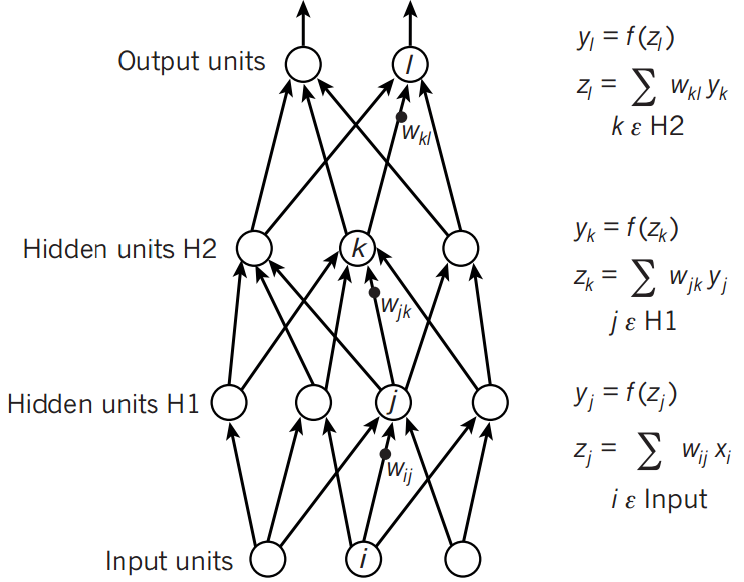如图所示，这里讲得已经很清楚了，前向传播的思想比较简单。
举个例子，假设上一层结点i,j,k,…等一些结点与本层的结点w有连接，那么结点w的值怎么算呢？就是通过上一层的i,j,k等结点以及对应的连接权值进行加权和运算，最终结果再加上一个偏置项（图中为了简单省略了），最后在通过一个非线性函数（即激活函数），如ReLu，sigmoid等函数，最后得到的结果就是本层结点w的输出。
最终不断的通过这种方法一层层的运算，得到输出层结果。
对于前向传播来说，不管维度多高，其过程都可以用如下公式表示：a2=σ(z2)=σ(a1∗W2+b2)a^2 = \sigma(z^2) = \sigma(a^1 * W^2 + b^2)
其中，上标代表层数，星号表示卷积，b表示偏置项bias，σ\sigma表示激活函数。
2.反向传播算法(Back propagation)
BackPropagation算法是多层神经网络的训练中举足轻重的算法。简单的理解，它的确就是复合函数的链式法则，但其在实际运算中的意义比链式法则要大的多。要回答题主这个问题“如何直观的解释back propagation算法？”  需要先直观理解多层神经网络的训练。
机器学习可以看做是数理统计的一个应用，在数理统计中一个常见的任务就是拟合，也就是给定一些样本点，用合适的曲线揭示这些样本点随着自变量的变化关系.
深度学习同样也是为了这个目的，只不过此时，样本点不再限定为(x, y)点对，而可以是由向量、矩阵等等组成的广义点对(X,Y)。而此时，(X,Y)之间的关系也变得十分复杂，不太可能用一个简单函数表示。然而，人们发现可以用多层神经网络来表示这样的关系，而多层神经网络的本质就是一个多层复合的函数。借用网上找到的一幅图，来直观描绘一下这种复合关系。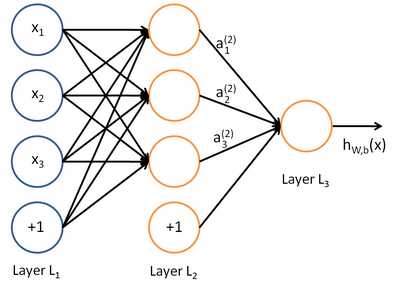其对应的表达式如下：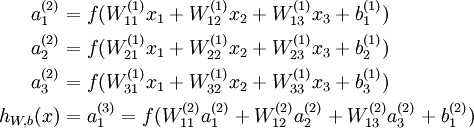上面式中的Wij就是相邻两层神经元之间的权值，它们就是深度学习需要学习的参数，也就相当于直线拟合y=k*x+b中的待求参数k和b。
和直线拟合一样，深度学习的训练也有一个目标函数，这个目标函数定义了什么样的参数才算一组“好参数”，不过在机器学习中，一般是采用成本函数（cost function），然后，训练目标就是通过调整每一个权值Wij来使得cost达到最小。cost函数也可以看成是由所有待求权值Wij为自变量的复合函数，而且基本上是非凸的，即含有许多局部最小值。但实际中发现，采用我们常用的梯度下降法就可以有效的求解最小化cost函数的问题。
梯度下降法需要给定一个初始点，并求出该点的梯度向量，然后以负梯度方向为搜索方向，以一定的步长进行搜索，从而确定下一个迭代点，再计算该新的梯度方向，如此重复直到cost收敛。那么如何计算梯度呢？
假设我们把cost函数表示为H(W11,W12,⋯ ,Wij,⋯ ,Wmn)H(W_{11}, W_{12}, \cdots , W_{ij}, \cdots, W_{mn}),那么它的梯度向量就等于∇H=∂H∂W11e11+⋯+∂H∂Wmnemn\nabla H  = \frac{\partial H}{\partial W_{11} }\mathbf{e}_{11} + \cdots + \frac{\partial H}{\partial W_{mn} }\mathbf{e}_{mn}, 其中eij\mathbf{e}_{ij}表示正交单位向量。为此，我们需求出cost函数H对每一个权值Wij的偏导数。而BP算法正是用来求解这种多层复合函数的所有变量的偏导数的利器。
我们以求e=(a+b)*(b+1)的偏导为例。
它的复合关系画出图可以表示如下：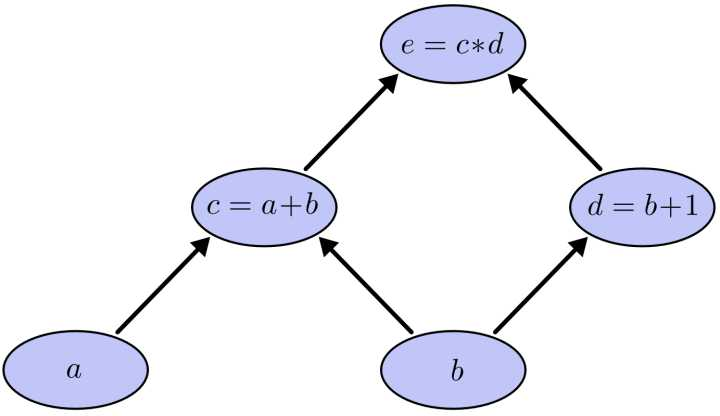在图中，引入了中间变量c,d。
为了求出a=2, b=1时，e的梯度，我们可以先利用偏导数的定义求出不同层之间相邻节点的偏导关系，如下图所示。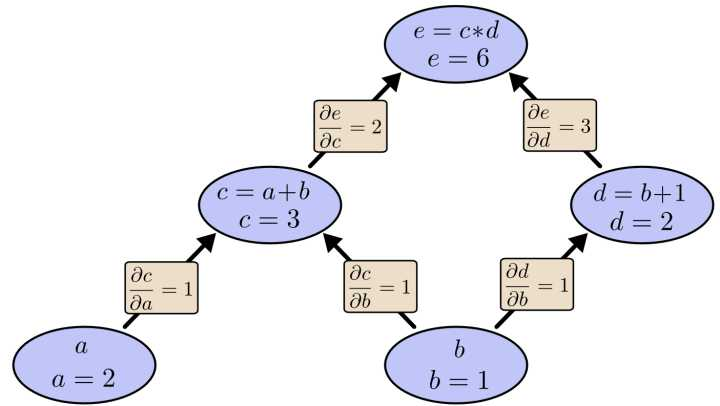利用链式法则我们知道：∂e∂a=∂e∂c⋅∂c∂a以及∂e∂b=∂e∂c⋅∂c∂b+∂e∂d⋅∂d∂b\frac{\partial e}{\partial a}=\frac{\partial e}{\partial c}\cdot \frac{\partial c}{\partial a}以及\frac{\partial e}{\partial b}=\frac{\partial e}{\partial c}\cdot \frac{\partial c}{\partial b}+\frac{\partial e}{\partial d}\cdot \frac{\partial d}{\partial b}
链式法则在上图中的意义是什么呢？其实不难发现，∂e∂a\frac{\partial e}{\partial a}的值等于从a到e的路径上的偏导值的乘积，而∂e∂b\frac{\partial e}{\partial b}的值等于从b到e的路径1(b-c-e)上的偏导值的乘积加上路径2(b-d-e)上的偏导值的乘积。也就是说，对于上层节点p和下层节点q，要求得∂p∂q\frac{\partial p}{\partial q}，需要找到从q节点到p节点的所有路径，并且对每条路径，求得该路径上的所有偏导数之乘积，然后将所有路径的 “乘积” 累加起来才能得到∂p∂q\frac{\partial p}{\partial q}的值。
大家也许已经注意到，这样做是十分冗余的，因为很多路径被重复访问了。比如上图中，a-c-e和b-c-e就都走了路径c-e。对于权值动则数万的深度模型中的神经网络，这样的冗余所导致的计算量是相当大的。
同样是利用链式法则，BP算法则机智地避开了这种冗余，它对于每一个路径只访问一次就能求顶点对所有下层节点的偏导值。
正如反向传播(BP)算法的名字说的那样，BP算法是反向(自上往下)来寻找路径的。
从最上层的节点e开始，初始值为1，以层为单位进行处理。对于e的下一层的所有子节点，将1乘以e到某个节点路径上的偏导值，并将结果“堆放”在该子节点中。等e所在的层按照这样传播完毕后，第二层的每一个节点都“堆放"些值，然后我们针对每个节点，把它里面所有“堆放”的值求和，就得到了顶点e对该节点的偏导。然后将这些第二层的节点各自作为起始顶点，初始值设为顶点e对它们的偏导值，以"层"为单位重复上述传播过程，即可求出顶点e对每一层节点的偏导数。
以上图为例，节点c接受e发送的12并堆放起来，节点d接受e发送的13并堆放起来，至此第二层完毕，求出各节点总堆放量并继续向下一层发送。节点c向a发送21并对堆放起来，节点c向b发送21并堆放起来，节点d向b发送31并堆放起来，至此第三层完毕，节点a堆放起来的量为2，节点b堆放起来的量为21+3*1=5, 即顶点e对b的偏导数为5.
举个不太恰当的例子，如果把上图中的箭头表示欠钱的关系，即c→e表示e欠c的钱。以a, b为例，直接计算e对它们俩的偏导相当于a, b各自去讨薪。a向c讨薪，c说e欠我钱，你向他要。于是a又跨过c去找e。b先向c讨薪，同样又转向e，b又向d讨薪，再次转向e。可以看到，追款之路，充满艰辛，而且还有重复，即a, b 都从c转向e。
而BP算法就是主动还款。e把所欠之钱还给c，d。c，d收到钱，乐呵地把钱转发给了a，b，皆大欢喜。
3.反向传播具体计算过程推导
为了方便起见，这里我定义了三层网络，输入层（第0层），隐藏层（第1层），输出层（第二层）。并且每个结点没有偏置（有偏置原理完全一样），激活函数为sigmod函数（不同的激活函数，求导不同），符号说明如下：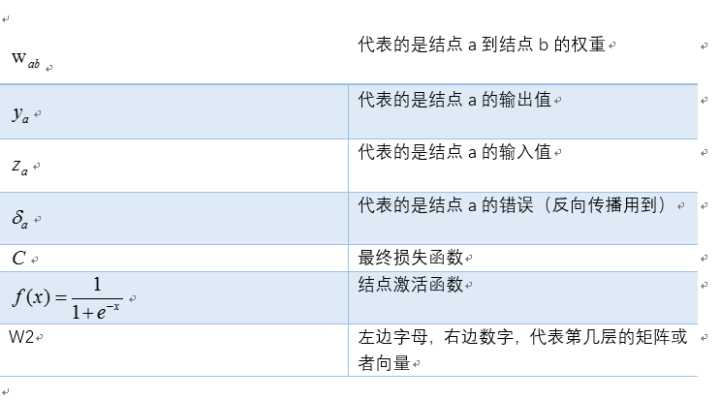对应网络如下：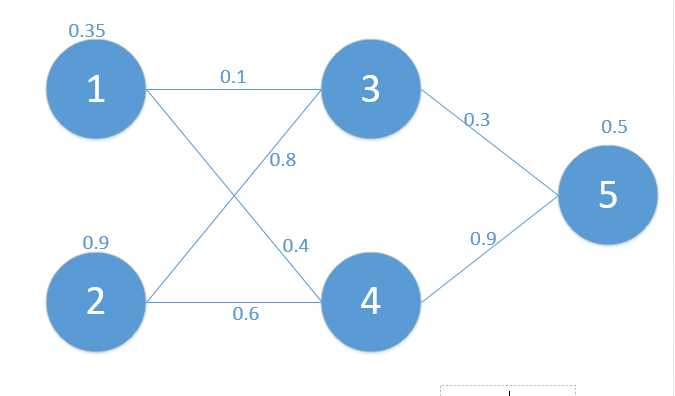其中对应的矩阵表示如下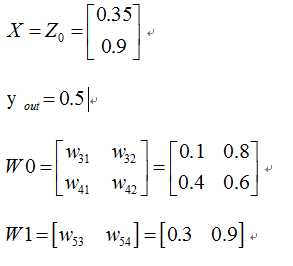首先我们先走一遍正向传播，公式与相应的数据对应如下：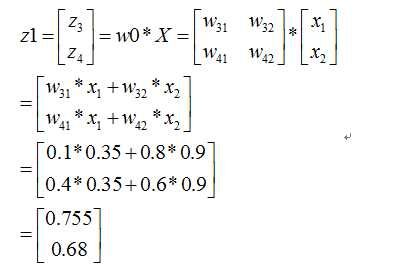那么：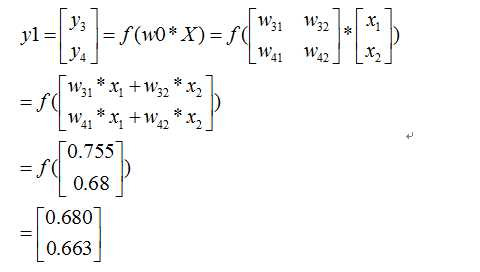同理可以得到：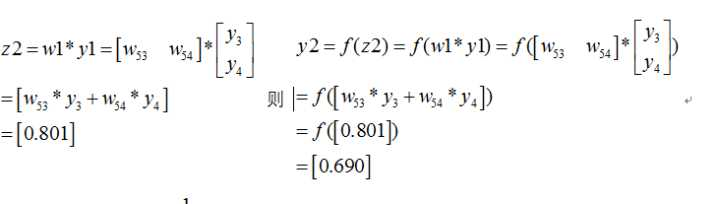那么最终的损失为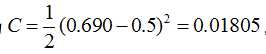，我们当然是希望这个值越小越好。这也是我们为什么要进行训练，调节参数，使得最终的损失最小。这就用到了我们的反向传播算法，实际上反向传播就是梯度下降法中链式法则的使用。
下面我们看如何反向传播
根据公式，我们有：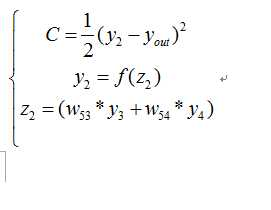这个时候我们需要求出C对w的偏导，则根据链式法则有：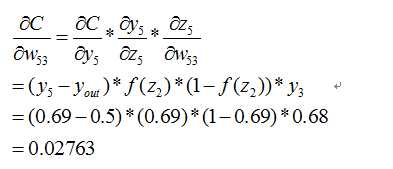同理有：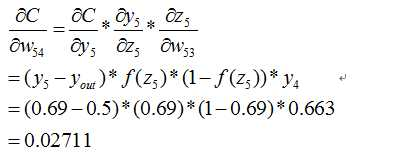到此我们已经算出了最后一层的参数偏导了.我们继续往前面链式推导：
我们现在还需要求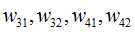，下面给出一个推导其它全都类似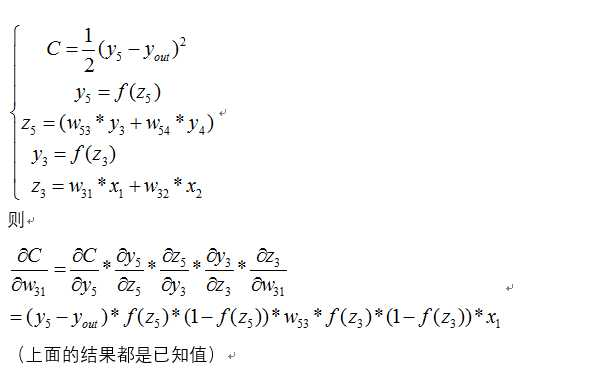同理可得其它几个式子：
则最终的结果为：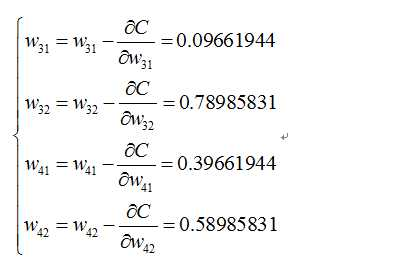再按照这个权重参数进行一遍正向传播得出来的Error为0.165
而这个值比原来的0.19要小，则继续迭代，不断修正权值，使得代价函数越来越小，预测值不断逼近0.5.我迭代了100次的结果，Error为5.92944818e-07（已经很小了，说明预测值与真实值非常接近了），最后的权值为：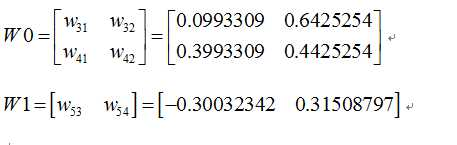bp过程可能差不多就是这样了，可能此文需要你以前接触过bp算法，只是还有疑惑，一步步推导后，会有较深的理解。
上面的python代码实现如下：
#!/usr/bin/env python
#coding:utf-8

import numpy as np

def nonlin(x, deriv = False):
if(deriv == True):
return x * (1 - x)
return 1 / (1 + np.exp(-x))

X = np.array([[0.35], [0.9]])
y = np.array([[0.5]])

np.random.seed(1)

W0 = np.array([[0.1, 0.8], [0.4, 0.6]])
W1 = np.array([[0.3, 0.9]])

print 'original ', W0, '\n', W1

for j in xrange(100):
l0 = X
l1 = nonlin(np.dot(W0, l0))
l2 = nonlin(np.dot(W1, l1))
l2_error = y - l2
Error = 1 / 2.0 * (y-l2)**2
print 'Error:', Error

l2_delta = l2_error * nonlin(l2, deriv=True)

l1_error = l2_delta * W1 #back propagation
l1_delta = l1_error * nonlin(l1, deriv=True)

W1 += l2_delta * l1.T
W0 += l0.T.dot(l1_delta)
print W0, '\n', W1

参考文档：
1.http://blog.csdn.net/lhanchao/article/details/51419150
2.https://www.zhihu.com/question/39022858


展开全文深度学习 反向传播
• ## PyTorch之前向传播函数forward

万次阅读 多人点赞 2018-11-14 11:37:40
3. 对输入进行处理（由定义的网络层进行处理）,主要体现在网络的前向传播； 4. 计算loss ，由Loss层计算； 5. 反向传播求梯度； 6. 根据梯度改变参数值,最简单的实现方式（SGD）为: weight = weight - learning_...
神经网络的典型处理如下所示：

1. 定义可学习参数的网络结构（堆叠各层和层的设计）；
2. 数据集输入；
3. 对输入进行处理（由定义的网络层进行处理）,主要体现在网络的前向传播；
4. 计算loss ，由Loss层计算；
5. 反向传播求梯度；
6. 根据梯度改变参数值,最简单的实现方式（SGD）为:
weight = weight - learning_rate * gradient

下面是利用PyTorch定义深度网络层（Op）示例：

class FeatureL2Norm(torch.nn.Module):
def __init__(self):
super(FeatureL2Norm, self).__init__()

def forward(self, feature):
epsilon = 1e-6
#        print(feature.size())
#        print(torch.pow(torch.sum(torch.pow(feature,2),1)+epsilon,0.5).size())
norm = torch.pow(torch.sum(torch.pow(feature,2),1)+epsilon,0.5).unsqueeze(1).expand_as(feature)
return torch.div(feature,norm)

class FeatureRegression(nn.Module):
def __init__(self, output_dim=6, use_cuda=True):
super(FeatureRegression, self).__init__()
self.conv = nn.Sequential(
nn.BatchNorm2d(128),
nn.ReLU(inplace=True),
nn.BatchNorm2d(64),
nn.ReLU(inplace=True),
)
self.linear = nn.Linear(64 * 5 * 5, output_dim)
if use_cuda:
self.conv.cuda()
self.linear.cuda()

def forward(self, x):
x = self.conv(x)
x = x.view(x.size(0), -1)
x = self.linear(x)
return x

由上例代码可以看到，不论是在定义网络结构还是定义网络层的操作（Op），均需要定义forward函数，下面看一下PyTorch官网对PyTorch的forward方法的描述：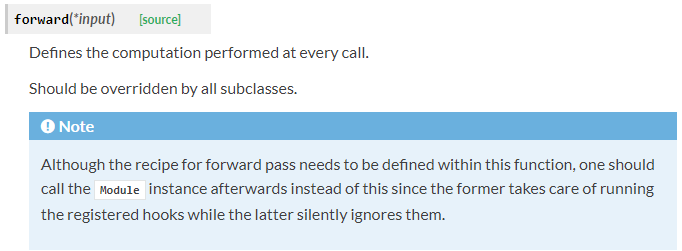那么调用forward方法的具体流程是什么样的呢？具体流程是这样的：

以一个Module为例：1. 调用module的call方法
2. module的call里面调用module的forward方法
3. forward里面如果碰到Module的子类，回到第1步，如果碰到的是Function的子类，继续往下
4. 调用Function的call方法
5. Function的call方法调用了Function的forward方法。
6. Function的forward返回值
7. module的forward返回值
8. 在module的call进行forward_hook操作，然后返回值。

上述中“调用module的call方法”是指nn.Module 的__call__方法。定义__call__方法的类可以当作函数调用，具体参考Python的面向对象编程。也就是说，当把定义的网络模型model当作函数调用的时候就自动调用定义的网络模型的forward方法。nn.Module 的__call__方法部分源码如下所示：

def __call__(self, *input, **kwargs):
result = self.forward(*input, **kwargs)
for hook in self._forward_hooks.values():
#将注册的hook拿出来用
hook_result = hook(self, input, result)
...
return result

可以看到，当执行model(x)的时候，底层自动调用forward方法计算结果。具体示例如下：

class LeNet(nn.Module):
def __init__(self):
super(LeNet, self).__init__()

layer1 = nn.Sequential()
self.layer1 = layer1

layer2 = nn.Sequential()
self.layer2 = layer2

layer3 = nn.Sequential()
self.layer3 = layer3
def forward(self, x):
x = self.layer1(x)
x = self.layer2(x)
x = x.view(x.size(0), -1)
x = self.layer3(x)
return x

model = LeNet()
y = model(x)

如上则调用网络模型定义的forward方法。

如果您觉得我的文章对您有所帮助，欢迎扫码进行赞赏！参考：

1. pytorch学习笔记（九）：PyTorch结构介绍

2. pytorch学习笔记（七）：pytorch hook 和 关于pytorch backward过程的理解

3. Pytorch入门学习（三）：Neural Networks


展开全文PyTorch forward
• 向传播和反向传播1. 前向传播2. 反向传播 例程：生产一批零件，将体积X1和重量X2为特征输入NN，通过NN后输出一个数值 1. 前向传播向传播过程 用Tensorflow表示前向传播 X为1*2的...
前向传播和反向传播1. 前向传播1.1  前向传播过程1.2  用Tensorflow表示前向传播1.3 实现神经网络前向传播过程，网络自动推理出输出 y 的值2. 反向传播2.1 损失函数2.2 反向传播训练方法：以减小 loss 值为优化目标
例程：生产一批零件，将体积X1和重量X2为特征输入NN，通过NN后输出一个数值
1. 前向传播

前向传播就是搭建模型的计算过程，让模型具有推理能力，可以针对一组输入给出相应的输出

1.1  前向传播过程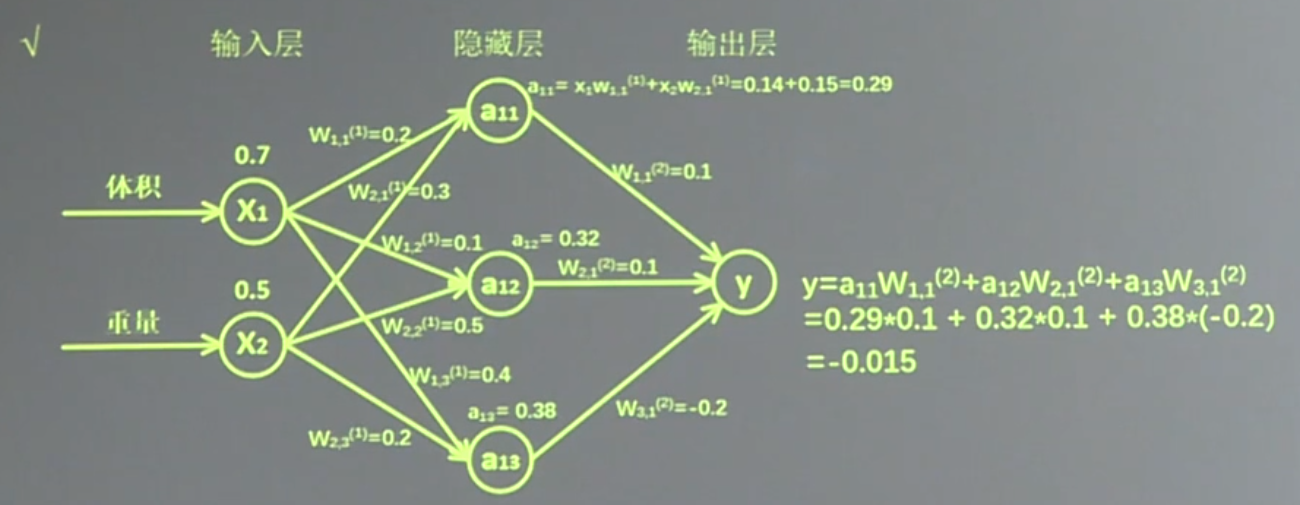第一层

X
$W^(1^)$
a

x 表示输入，是一个 1 行 2 列矩阵，表示1次输入1组特征(包含了体积和重量两个元素)
对于第一层的 w 前面有两个节点,后面有三个节点 $W^(1^)$ 应该是个2行3列矩阵
a 为第一层网络，a 是一个1行3列矩阵

第二层

$W^(2^)$

参数要满足前面三个节点，后面一个节点，所以 是3行1列矩阵

注：神经网络共有几层（或当前是第几层网络）都是指的计算层，输入不是计算层，所以 a 为第一层网络
1.2  用Tensorflow表示前向传播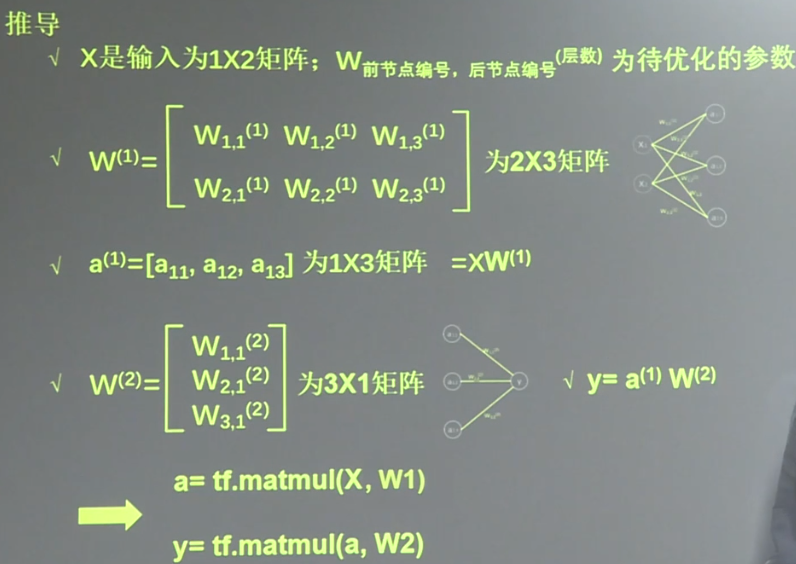变量初始化、计算图节点运算都要用会话（with 结构）实现
with tf.Session() as sess:
sess.run()

变量初始化：在 sess.run 函数中用 tf.global_variables_initializer()汇总所有待优化变量。
init_op = tf.global_variables_initializer()
sess.run(init_op)
计算图节点运算：在 sess.run 函数中写入待运算的节点
sess.run(y)
用 tf.placeholder 占位，在 sess.run 函数中用 feed_dict 喂数据
喂一组数据：
x = tf.placeholder(tf.float32, shape=(1, 2))
sess.run(y, feed_dict={x: [[0.5,0.6]]})
喂多组数据：
x = tf.placeholder(tf.float32, shape=(None, 2))
sess.run(y, feed_dict={x: [[0.1,0.2],[0.2,0.3],[0.3,0.4],[0.4,0.5]]})

1.3 实现神经网络前向传播过程，网络自动推理出输出 y 的值

例1：用 placeholder 实现输入定义（sess.run 中喂入一组数据）的情况
第一组喂体积 0.7、重量 0.5

#coding:utf-8
import tensorflow as tf

#定义输入和参数
x=tf.placeholder(tf.float32,shape=(1,2))
w1=tf.Variable(tf.random_normal([2,3],stddev=1,seed=1))
w2=tf.Variable(tf.random_normal([3,1],stddev=1,seed=1))

#定义前向传播过程
a=tf.matmul(x,w1)
y=tf.matmul(a,w2)

#用会话计算结果
with tf.Session() as sess:
init_op=tf.global_variables_initializer()
sess.run(init_op)
print (sess.run(y,feed_dict={x:[[0.7,0.5]]}))


例2：用 placeholder 实现输入定义（sess.run 中喂入多组数据）的情况
第一组喂体积 0.7、重量 0.5，第二组喂体积 0.2、重量 0.3，第三组喂体积0.3、重量 0.4，第四组喂体积 0.4、重量 0.5.

#coding:utf-8
import tensorflow as tf
#定义输入和参数
x=tf.placeholder(tf.float32,shape=(None,2))
w1=tf.Variable(tf.random_normal([2,3],stddev=1,seed=1))
w2=tf.Variable(tf.random_normal([3,1],stddev=1,seed=1))
#定义前向传播过程
a=tf.matmul(x,w1)
y=tf.matmul(a,w2)
#用会话计算结果
with tf.Session() as sess:
init_op=tf.global_variables_initializer()
sess.run(init_op)
print(sess.run(y,feed_dict={x:[[0.7,0.5],[0.2,0.3],[0.3,0.4],[0.4,0.5]]}))

2. 反向传播

反向传播：就是训练模型参数，在所有参数上用梯度下降，使 NN 模型在训练数据上的损失函数最小

2.1 损失函数

损失函数（loss）：计算得到的预测值 y 与已知答案 y_的差距

损失函数计算方法：有很多方法，均方误差 MSE 是比较常用的方法之一

均方误差 MSE
解释

定义
求前向传播计算结果与已知答案之差的平方再求平均

公式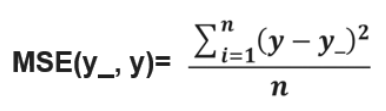tensorflow 函数表示
loss_mse = tf.reduce_mean(tf.square(y_ - y))

2.2 反向传播训练方法：以减小 loss 值为优化目标

反向传播训练方法
梯度下降
momentum 优化器
、、、

用 tensorflow 函数表示
train_step=tf.train.MomentumOptimizer(learning_rate, momentum).minimize(loss)

区别
使用随机梯度下降算法，使参数沿着梯度的反方向，即总损失减小的方向移动，实现更新参数
更新参数时，利用了超参数

参数更新的公式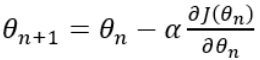损失函数：J(?)  参数：?，学习率：?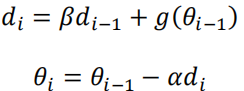?为学习率，超参数为?，?为参数，损失函数的梯度：?(??−1)
无

学习率

决定每次参数更新的幅度
优化器中都需要一个叫做学习率的参数，使用时，如果学习率选择过大会出现震荡不收敛的情况，如果学习率选择过小，会出现收敛速度慢的状况。我们可以选个比较小的值填入，比如 0.01、0.001


展开全文• 文章目录一、深度神经网络（DNN）模型1.1 从感知机到神经网络1.2 DNN的基本结构二、DNN前向传播算法2.1 DNN前向传播算法数学原理2.2 DNN前向传播算法2.3 DNN前向传播算法小结三、DNN反向传播算法3.1 DNN反向传播算法...
文章目录一、深度神经网络（DNN）模型1.1 从感知机到神经网络1.2 DNN的基本结构二、DNN前向传播算法2.1 DNN前向传播算法数学原理2.2 DNN前向传播算法2.3 DNN前向传播算法小结三、DNN反向传播算法3.1 DNN反向传播算法要解决的问题3.2 DNN反向传播算法的基本思路3. 3 DNN反向传播算法过程3.4 DNN反向传播算法小结参考文献
一、深度神经网络（DNN）模型
深度神经网络（Deep Neural Networks， 以下简称DNN）是深度学习的基础，而要理解DNN，首先我们要理解DNN模型，下面我们就对DNN的模型与前向传播算法做一个总结。
1.1 从感知机到神经网络
感知机的模型大家都比较熟悉，它是一个有若干输入和一个输出的模型，如下图: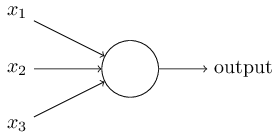输出和输入之间学习到一个线性关系，得到中间输出结果：
$z=\sum\limits_{i=1}^mw_ix_i + b$
接着是一个神经元激活函数:
$sign(z)= \begin{cases} -1& {z<0}\\ 1& {z\geq 0} \end{cases}$
从而得到我们想要的输出结果1或者-1。
这个模型只能用于二元分类，且无法学习比较复杂的非线性模型，因此在工业界无法使用。
而神经网络则在感知机的模型上做了扩展，总结下主要有三点：
1）加入了隐藏层，隐藏层可以有多层，增强模型的表达能力，如下图实例，当然增加了这么多隐藏层模型的复杂度也增加了好多。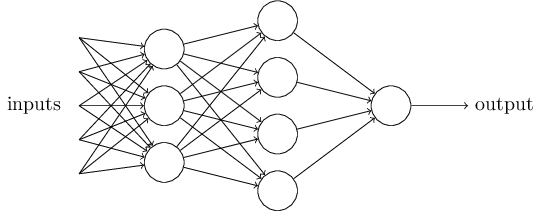2）输出层的神经元也可以不止一个输出，可以有多个输出，这样模型可以灵活的应用于分类回归，以及其他的机器学习领域比如降维和聚类等。多个神经元输出的输出层对应的一个实例如下图，输出层现在有4个神经元了。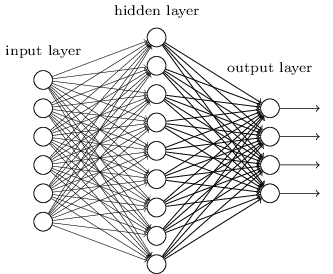3） 对激活函数做扩展，感知机的激活函数是$sign(z)$，虽然简单但是处理能力有限，因此神经网络中一般使用的其他的激活函数，比如我们在逻辑回归里面使用过的Sigmoid函数，即：
$f(z)=\frac{1}{1+e^{-z}}$
还有后来出现的tanx, softmax,和ReLU等。通过使用不同的激活函数，神经网络的表达能力进一步增强。对于各种常用的激活函数，我随后会整理一份资料。
1.2 DNN的基本结构
上一节我们了解了神经网络基于感知机的扩展，而DNN可以理解为有很多隐藏层的神经网络。这个很多其实也没有什么度量标准, 多层神经网络和深度神经网络DNN其实也是指的一个东西，当然，DNN有时也叫做多层感知机（Multi-Layer perceptron,MLP）, 名字实在是多。后面我们讲到的神经网络都默认为DNN。
从DNN按不同层的位置划分，DNN内部的神经网络层可以分为三类，输入层，隐藏层和输出层，如下图示例，一般来说第一层是输入层，最后一层是输出层，而中间的层数都是隐藏层。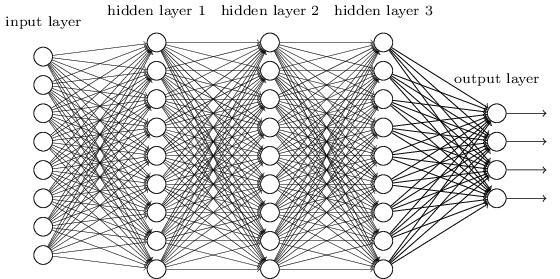层与层之间是全连接的，也就是说，第$i$层的任意一个神经元一定与第$i+1$层的任意一个神经元相连。虽然DNN看起来很复杂，但是从小的局部模型来说，还是和感知机一样，即一个线性关系$z=\sum\limits w_ix_i + b$ 加上一个激活函数$\sigma(z)$。
由于DNN层数多，则我们的线性关系系数$w$和偏倚$b$的数量也就是很多了。具体的参数在DNN是如何定义的呢？
首先我们来看看线性关系系数$w$的定义。以下图一个三层的DNN为例，第二层的第4个神经元到第三层的第2个神经元的线性系数定义为$w_{24}^3$。上标3代表线性系数$w$所在的层数，而下标对应的是输出的第三层索引2和输入的第二层索引4。你也许会问，为什么不是$w_{42}^3$, 而是$w_{24}^3$呢？这主要是为了便于模型用于矩阵表示运算，如果是$w_{42}^3$每次进行矩阵运算是$w^Tx+b$，需要进行转置。将输出的索引放在前面的话，则线性运算不用转置，即直接为$wx+b$。总结下，第$l−1$层的第$k$个神经元到第$l$层的第$j$个神经元的线性系数定义为$w_{jk}^l$。注意，输入层是没有$w$参数的。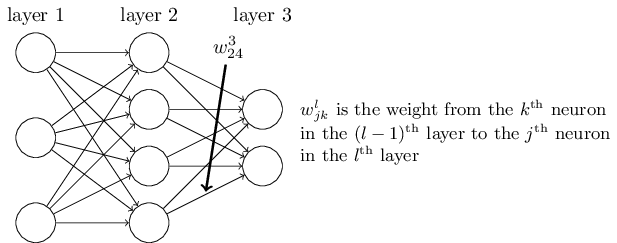再来看看偏倚$b$的定义。还是以这个三层的DNN为例，第二层的第3个神经元对应的偏倚定义为$b_3^{2}$。其中，上标2代表所在的层数，下标3代表偏倚所在的神经元的索引。输入层是没有偏倚参数$b$的。
同样的道理，对于神经元的激活值而言，第3层的第1个神经元的激活值应该表示为$a_1^{3}$。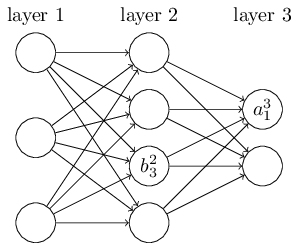二、DNN前向传播算法
2.1 DNN前向传播算法数学原理
在上一节，我们已经介绍了DNN各层线性关系系数$w$和偏倚$b$的定义。假设我们选择的激活函数是$\sigma(z)$，隐藏层和输出层的输出值为$a$，则对于下图的三层DNN，利用和感知机一样的思路，我们可以利用上一层的输出计算下一层的输出，也就是所谓的DNN前向传播算法。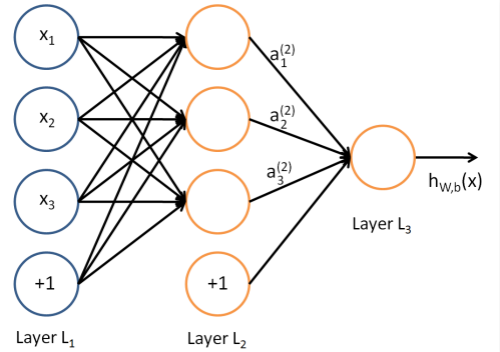对于第二层的的输出$a_1^2,a_2^2,a_3^2$，我们有：
$a_1^2=\sigma(z_1^2) = \sigma(w_{11}^2x_1 + w_{12}^2x_2 + w_{13}^2x_3 + b_1^{2})$
$a_2^2=\sigma(z_2^2) = \sigma(w_{21}^2x_1 + w_{22}^2x_2 + w_{23}^2x_3 + b_2^{2})$
$a_3^2=\sigma(z_3^2) = \sigma(w_{31}^2x_1 + w_{32}^2x_2 + w_{33}^2x_3 + b_3^{2})$
对于第三层的的输出$a_1^3$，我们有：
$a_1^3=\sigma(z_1^3) = \sigma(w_{11}^3a_1^2 + w_{12}^3a_2^2 + w_{13}^3a_3^2 + b_1^{3})$
将上面的例子一般化，假设第$l−1$层共有$m$个神经元，则对于第$l$层的第$j$个神经元的输出$a_j^l$，我们有：
$a_j^l = \sigma(z_j^l) = \sigma(\sum\limits_{k=1}^mw_{jk}^la_k^{l-1} + b_j^l)$
其中，如果$l=2$，则对于的$a_k^1$即为输入层的$x_k$。
从上面可以看出，使用代数法一个个的表示输出比较复杂，而如果使用矩阵法则比较的简洁。假设第$l−1$层共有$m$个神经元，而第$l$层共有$n$个神经元，则第$l$层的线性系数$w$组成了一个$n×m$的矩阵$W^l$, 第$l$层的偏倚$b$组成了一个$n×1$的向量$b^l$ , 第$l−1$层的输出$a$组成了一个$m×1$的向量$a^{l−1}$，第$l$层的未激活前线性输出$z$组成了一个$n×1$的向量$z^l$, 第$l$层的的输出$a$组成了一个$n×1$的向量$a^l$。则用矩阵法表示，第$l$层的输出为：
$a^l = \sigma(z^l) = \sigma(W^la^{l-1} + b^l)$
这个表示方法简洁漂亮，后面我们的讨论都会基于上面的这个矩阵法表示来。所以，应该时刻记住我们符号的含义，否则在后面推导反向传播公式时会比较懵。
2.2 DNN前向传播算法
有了上一节的数学推导，DNN的前向传播算法也就不难了。所谓的DNN的前向传播算法也就是利用我们的若干个权重系数矩阵$W$和偏倚向量$b$来和输入值向量$x$进行一系列线性运算和激活运算，从输入层开始，一层层的向后计算，一直到运算到输出层，得到输出结果为止 。
输入: 总层数$L$，所有隐藏层和输出层对应的矩阵$W$，偏倚向量$b$，输入值向量$x$
输出：输出层的输出$a^L$

1） 初始化$a^1=x$

2） for $l=2$ to $L$, 计算：

$a^l = \sigma(z^l) = \sigma(W^la^{l-1} + b^l)$
最后的结果即为输出$a^L$。
2.3 DNN前向传播算法小结
单独看DNN前向传播算法，似乎没有什么大用处，而且这一大堆的矩阵$W$,偏倚向量$b$对应的参数怎么获得呢？怎么得到最优的矩阵$W$,偏倚向量$b$呢？这个我们在下一章讲DNN的反向传播算法时再讲。而理解反向传播算法的前提就是理解DNN的模型与前向传播算法。这也是我们先讲前向传播算法的原因。
三、DNN反向传播算法
3.1 DNN反向传播算法要解决的问题
在了解DNN的反向传播算法（Back Propagation，BP）前，我们先要知道DNN反向传播算法要解决的问题，也就是说，什么时候我们需要这个反向传播算法？
回到我们监督学习的一般问题，假设我们有$m$个训练样本：$\{(x_1,y_1), (x_2,y_2), ..., (x_m,y_m)\}$，其中$x$为输入向量，特征维度为$n_{in}$，而$y$为输出向量，特征维度为$n_{out}$。我们需要利用这$m$个样本训练出一个模型，当有一个新的测试样本$(x_{test},?)$来到时, 我们可以预测$y_{test}$向量的输出。
如果我们采用DNN的模型，即我们使输入层有$n_{in}$个神经元，而输出层有$n_{out}$个神经元。再加上一些含有若干神经元的隐藏层。此时我们需要找到合适的所有隐藏层和输出层对应的线性系数矩阵$W$和偏倚向量$b$，让所有的训练样本输入计算出的输出尽可能的等于或很接近样本输出。怎么找到合适的参数呢？
如果大家对传统的机器学习的算法优化过程熟悉的话，这里就很容易联想到我们可以用一个合适的损失函数来度量训练样本的输出损失，接着对这个损失函数进行优化求最小化的极值，对应的一系列线性系数矩阵$W$和偏倚向量$b$即为我们的最终结果。在DNN中，损失函数优化极值求解的过程最常见的一般是通过梯度下降法来一步步迭代完成的，当然也可以是其他的迭代方法比如牛顿法与拟牛顿法。
对DNN的损失函数用梯度下降法进行迭代优化求极小值的过程即为我们的反向传播算法。
3.2 DNN反向传播算法的基本思路
在进行DNN反向传播算法前，我们需要选择一个损失函数，来度量训练样本计算出的输出和真实的训练样本输出之间的损失。你也许会问：训练样本计算出的输出是怎么得来的？这个输出是随机选择一系列$W,b$，用前向传播算法计算出来的。即通过一系列的计算：$a^l = \sigma(z^l) = \sigma(W^la^{l-1} + b^l)$。计算到输出层第$L$层对应的$a^L$即为前向传播算法计算出来的输出。
回到损失函数，DNN可选择的损失函数有不少，为了专注算法，这里我们使用最常见的均方差来度量损失。当然，针对不同的任务，可以选择不同的损失函数。即对于每个样本，我们期望最小化下式：
$J(W,b,x,y) = \frac{1}{2}||a^L-y||_2^2$
其中，$a^L$和$y$为特征维度为$n_{out}$的向量，而$||S||_2$为$S$的L2范数。
损失函数有了，现在我们开始用梯度下降法迭代求解每一层的$W,b$。
注：以下是BP算法推导的过程，是本文最核心，也是神经网络最基本的公式推导。
思路第一步：
首先是输出层第$L$层。注意到输出层的$W,b$满足下式：
$a^L = \sigma(z^L) = \sigma(W^La^{L-1} + b^L) \qquad \text{(1)}$
这样对于输出层的参数，我们的损失函数变为：
$J(W,b,x,y) = \frac{1}{2}||a^L-y||_2^2 = \frac{1}{2}|| \sigma(W^La^{L-1} + b^L)-y||_2^2 \qquad \text{(2)}$
这样求解$W,b$的梯度就简单了：
$\frac{\partial J(W,b,x,y)}{\partial W^L} = \frac{\partial J(W,b,x,y)}{\partial z^L}\frac{\partial z^L}{\partial W^L} =\frac{\partial J(W,b,x,y)}{\partial a^L}\frac{\partial a^L}{\partial z^L}\frac{\partial z^L}{\partial W^L} =(a^L-y) \odot \sigma^{'}(z^L)(a^{L-1})^T\qquad \text{(3)}$
$\frac{\partial J(W,b,x,y)}{\partial b^L} = \frac{\partial J(W,b,x,y)}{\partial z^L}\frac{\partial z^L}{\partial b^L} =\frac{\partial J(W,b,x,y)}{\partial a^L}\frac{\partial a^L}{\partial z^L}\frac{\partial z^L}{\partial b^L}=(a^L-y)\odot \sigma^{'}(z^L)\qquad \text{(4)}$
注意上式中有一个符号$\odot$,它代表Hadamard积，对于两个维度相同的向量$A（a_1,a_2,...a_n）^T$和$B（b_1,b_2,...b_n）^T$，则$A \odot B = (a_1b_1, a_2b_2,...a_nb_n)^T$。

对于公式（3）和（4），我在这里多解释一下为什么是这样：
对于公式（3）：前两项之所以是Hadamard积的形式，是因为$\frac{\partial J(W,b,x,y)}{\partial a^L}\frac{\partial a^L}{\partial z^L}$都是针对同一层的神经元。如果我们考虑对于$L$层的第$j$个神经元，即$\frac{\partial J(W,b,x,y)}{\partial a_j^L}\sigma^{'}(z_j^L)$，那么整合这一层的神经元，自然是$(a^L-y) \odot \sigma^{'}(z^L)$这样Hadamard积的形式。那么$(a^{L-1})^T$为什么在（3）中的最后呢？这涉及到矩阵求导的知识，不是我们本文的重点。在这里，用到的知识是：如果
$Y=WX+B$
那么
$\frac{\partial C}{\partial W}=\frac{\partial C}{\partial Y}X^T$
这样，即可推出公式（3）。公式（4）与公式（3）类似。

思路第二步：
我们注意到在求解输出层的$W,b$的时候，有公共的部分$\frac{\partial J(W,b,x,y)}{\partial z^L}$，因此我们可以把公共的部分即对$z^L$先算出来，记为：
$\delta^L = \frac{\partial J(W,b,x,y)}{\partial z^L} = (a^L-y)\odot \sigma^{'}(z^L) \qquad \text{(5)}$
根据公式（3）（4），我们可以把输出层的梯度算出来，那么如何计算上一层$L−1$层的梯度，上上层$L−2$层的梯度呢？这里我们需要一步步的递推，注意到对于第$l$层的未激活输出$z^l$，它的梯度可以表示为:
$\delta^l =\frac{\partial J(W,b,x,y)}{\partial z^l} = \frac{\partial J(W,b,x,y)}{\partial z^L}\frac{\partial z^L}{\partial z^{L-1}}\frac{\partial z^{L-1}}{\partial z^{L-2}}...\frac{\partial z^{l+1}}{\partial z^{l}}\qquad \text{(6)}$
如果我们可以依次计算出第$l$层的$\delta^l$,则该层的$W^l,b^l$很容易计算？为什么呢？注意到根据前向传播算法，我们有：
$z^l= W^la^{l-1} + b^l\qquad \text{(7)}$
所以根据上式我们可以很方便的计算出第$l$层的$W_l,b_l$的梯度如下：
$\frac{\partial J(W,b,x,y)}{\partial W^l} = \frac{\partial J(W,b,x,y)}{\partial z^l} \frac{\partial z^l}{\partial W^l} = \delta^{l}(a^{l-1})^T\qquad \text{(8)}$
$\frac{\partial J(W,b,x,y)}{\partial b^l} = \frac{\partial J(W,b,x,y)}{\partial z^l} \frac{\partial z^l}{\partial b^l} = \delta^{l}\qquad \text{(9)}$
思路第三步：
那么现在问题的关键就是要求出$\delta^l$了。这里我们用数学归纳法，第$L$层的$\delta^L$上面我们已经求出， 假设第$l+1$层的$\delta^{l+1}$已经求出来了，那么我们如何求出第$l$层的$\delta^l$呢？我们注意到：
$\delta^{l} = \frac{\partial J(W,b,x,y)}{\partial z^l} = \frac{\partial J(W,b,x,y)}{\partial z^{l+1}}\frac{\partial z^{l+1}}{\partial z^{l}} = \delta^{l+1}\frac{\partial z^{l+1}}{\partial z^{l}}\qquad \text{(10)}$
可见，用归纳法递推$\delta^{l+1}$和$\delta^l$的关键在于求解$\frac{\partial z^{l+1}}{\partial z^{l}}$。
而$z^{l+1}$和$z^l$的关系其实很容易找出：
$z^{l+1}= W^{l+1}a^{l} + b^{l+1} = W^{l+1}\sigma(z^l) + b^{l+1} \qquad \text{(11)}$
这样很容易求出：
$\frac{\partial z^{l+1}}{\partial z^{l}} = (W^{l+1})^T\odot \underbrace{(\sigma^{'}(z^l),..,\sigma^{'}(z^l))}_{n_{l+1}}\qquad \text{(12)}$
公式（12）的意思就是$(W^{l+1})^T$的每一列都Hadamard积$\sigma^{'}(z^l)$。
将公式（12）代入公式（10）我们得到：
$\delta^{l} = \delta^{l+1}\frac{\partial z^{l+1}}{\partial z^{l}} =( (W^{l+1})^T\delta^{l+1})\odot \sigma^{'}(z^l)\qquad \text{(13)}$

公式（13）的推导过程为：
$\delta^{l+1}\frac{\partial z^{l+1}}{\partial z^{l}} =\frac{\partial (\delta^{l+1})^T z^{l+1}}{\partial z^{l}} = \frac{\partial (\delta^{l+1})^T (W^{l+1}\sigma(z^l) + b^{l+1})}{\partial z^{l}} = \frac{\partial (\delta^{l+1})^T W^{l+1}\sigma(z^l) }{\partial z^{l}} \\= ((\delta^{l+1})^T W^{l+1})^T\odot \sigma^{'}(z^l) = = ((W^{l+1})^T\delta^{l+1})\odot \sigma^{'}(z^l)$

现在我们得到了$\delta^l$的递推关系式，只要求出了某一层的$\delta^l$，求解$W^l,b^l$的对应梯度就很简单。
总结：
其实，对于更新每一层的$W^l,b^l$的对应梯度，我们仔细观察整个过程，发现只需要四个公式就可以完整地进行更新。这就是著名的反向传播的四个公式，即公式(5)、(13)、(8)、(9)。我们稍加改动，使其可以应用到多种损失函数，即：
\begin{aligned} \delta^L = \frac{\partial J}{\partial a^L} \odot \sigma^{'}(z^L) \qquad \text{(BP1)}\\ \delta^{l} = (W^{l+1})^T\delta^{l+1}\odot \sigma^{'}(z^l)\qquad \text{(BP2)}\\ \frac{\partial J}{\partial W^l} = \delta^{l}(a^{l-1})^T\qquad \text{(BP3)}\\ \frac{\partial J}{\partial b^l} = \delta^{l}\qquad \text{(BP4)} \end{aligned}
3. 3 DNN反向传播算法过程
现在我们总结下DNN反向传播算法的过程。由于梯度下降法有批量（Batch），小批量(mini-Batch)，随机三个变种，为了简化描述，这里我们以最基本的批量梯度下降法为例来描述反向传播算法。实际上在业界使用最多的是mini-Batch的梯度下降法。不过区别仅仅在于迭代时训练样本的选择而已。
输入: 总层数$L$，以及各隐藏层与输出层的神经元个数，激活函数，损失函数，迭代步长$\alpha$，最大迭代次数MAX与停止迭代阈值$\epsilon$，输入的$m$个训练样本$\{(x_1,y_1), (x_2,y_2), ..., (x_m,y_m)\}$
输出：各隐藏层与输出层的线性关系系数矩阵$W$和偏倚向量$b$
1）初始化各隐藏层与输出层的线性关系系数矩阵$W$和偏倚向量$b$的值为一个随机值。
2）for iter to 1 to MAX：
2-1) for $i =1$ to $m$：
a) 将DNN输入$a^1$设置为$x_i$
b) for $l=2$ to $L$，进行前向传播算法计算$a^{i,l} = \sigma(z^{i,l}) = \sigma(W^la^{i,l-1} + b^l)$
c) 通过损失函数计算输出层的$\delta^{i,L}$（BP1）
d) for $l= L-1$ to $2$, 进行反向传播算法计算$\delta^{i,l} = (W^{l+1})^T\delta^{i,l+1}\odot \sigma^{'}(z^{i,l})$（BP2）
2-2) for $l = 2$ to $L$，更新第$l$层的$W^l,b^l$:
$W^l = W^l -\alpha \sum\limits_{i=1}^m \delta^{i,l}(a^{i, l-1})^T \qquad \text{(BP3)}$
$b^l = b^l -\alpha \sum\limits_{i=1}^m \delta^{i,l} \qquad \text{(BP4)}$
2-3) 如果所有$W$，$b$的变化值都小于停止迭代阈值$ϵ$，则跳出迭代循环到步骤3。
3） 输出各隐藏层与输出层的线性关系系数矩阵$W$和偏倚向量$b$。
注：上述的算法其实看起来有些复杂了，而实际上根据机器学习里普通的批梯度下降算法，我们在求出$m$个样本的$\delta^{i,L}$后，求平均得到$\delta^{L}$，然后反向继续更新$\delta^{l}$。
3.4 DNN反向传播算法小结
有了DNN反向传播算法，我们就可以很方便的用DNN的模型去解决各种监督学习的分类回归问题。当然DNN的参数众多，矩阵运算量也很大，直接使用会有各种各样的问题。有哪些问题以及如何尝试解决这些问题并优化DNN模型与算法，我们会在其它博客里讲。
参考文献
【1】深度神经网络（DNN）模型与前向传播算法
【2】深度神经网络（DNN）反向传播算法(BP)
本文转自【1】【2】
【3】Neural Networks and Deep Learning
【4】数学-矩阵计算（4）两种布局
【5】Matrix calculus
【6】反向传播四：反向传播的4个公式
【7】机器学习中的矩阵、向量求导


展开全文反向传播BP 神经网络
• 今天重温后向传播算法的推导，但重要的是比较前向传播和后向传播的优缺点，以及它们在神经网络中起到了什么不一般的作用，才让我们如此着迷。反向传播的由来反向传播由Hinton在1986年发明，该论文发表在
• 详解神经网络的前向传播和反向传播 本篇博客是对Michael Nielsen所著的《Neural Network and Deep Learning》第2章内容的解读，有兴趣的朋友可以直接阅读原文Neural Network and Deep Learning。 　对神经网络有些...推导
• ## 传染病传播模型（含matlab代码）

万次阅读 多人点赞 2020-01-25 19:33:10
随着卫生设施的改善、医疗水平的提高以及人类文明的不断发展,诸如霍乱、天花等曾经肆虐全球的传染性疾病已经得到有效的控制，但是一些新的、不断变异着的传染病毒却悄悄人类袭来。长期以来，建立数学模型以描述...matlab 数学建模
• 最近在看神经网络中的前向传播算法(FP)和反向传播算法(BP)，特地进行总结一下，方便以后理解。1.基本概念  上图是一张自己画的神经网络的图。假设每一层都添加了偏度单元（即值为1的神经元），用来表示阀值（因为...BP算法 神经网络
• 向传播过程 在讨论反向传播之前，我们讨论一下前向传播，即根据输入X来计算输出Y。输入X用矩阵表示，我们看一下如何基于矩阵X来计算网络的输出Y。 我们使用 表示从层的第 个神经元到层的个神经元的链接上的权重...
• 传播误差 简介 II . 输出层误差计算公式 III . 隐藏层层误差计算公式 IV . 使用误差更新 连接权值 V . 使用误差更新 单元偏置 VI . 反向传播 过程 VII . 损失函数 简介 VIII . 损失函数 IX . 损失函数 举例 X ....数据挖掘 神经网络 损失函数 反向传播
• ## TensorFlow2 手把手教你实现前向传播

千次阅读 多人点赞 2021-06-09 06:00:33
TensorFlow2 手把手教你实现前向传播.
• 所以反向传播算法是，先进行前向传播计算a(1),a(2)的值，存储在内存中。然后从更新输出层到倒数第一个隐藏层之间的权重，将计算的梯度保存到内存中，再更新倒数第一个隐藏层到倒数第二个隐藏层之间的梯度，这个...
• Back Propagation后向传播算法 Python实现后向传播算法用来自动调节神经网络的参数，本次实现代码完全参照Back Propagation的讲解 选取sklearn.datasets的moon数据集的前250个样本进行训练，迭代10000次之后可以...python 机器学习 神经网络
• 向传播 输入，输出，缓存为 前向传播的过程： 向量化的过程可以写成： 式中，就是初始的输入X。如下图的两层神经网络所示，可以很好的理解。 反向传播 反向传播主要采用数学上的链式法则，反向...
• 二是整理前馈神经网络中正向传播、误差反向传播和梯度下降的原理；三是梯度消失和梯度爆炸问题的原因及解决思路。 一、神经网络结构 目前比较常用的神经网络结构有如下三种： 1、前馈神经网络 前馈神经网络...误差反向传播
• 循环网络的前向传播算法非常简单，对于t时刻： 其中ϕ(.)为激活函数，一般来说会选择tanh函数，b为偏置。则 t 时刻的输出： 最终模型的预测输出为： 其中σ为激活函数，激活函数通常选择softmax...RNN LSTM
• 本文只包含CNN的前向传播和反向传播，主要是卷积层和pool层的前向传播和反向传播，一些卷积网络的基础知识不涉及 符号表示 如果lll层是卷积层： p[l]p[l]p^{[l]}: padding s[l]s[l]s^{[l]}: stride n[l]cnc...
• 向传播算法 Forward propagation 与反向传播算法 Back propagation神经网络 反向传播 深度学习 python
• ## 前向传播和反向传播（举例说明）

万次阅读 多人点赞 2017-09-19 16:25:06
假设神经网络结构如下图所示：有2个...----前向传播---- 隐含层神经元h1的输入： 代入数据可得： 假设激励函数用logistic函数，计算得隐含层神经元h1的输出： 同样的方法，可以得到隐含层神经元h
• ## 前向传播算法

千次阅读 2017-07-10 20:01:59
一个神经元有多个输入和一个输出，每个神经元的输入既可以是其他神经元的输出也可以是整个神经网络的输入。所谓神经网络的结构就是指的是不同的神经元之间的连接结构。 如图所示，一个最简单的神经元结构的输出就是...
• 在神经网络模型中包括前向传播和反向传播那么究竟什么是前向传播什么是反向传播呢前向传播：说的通俗一点就是从输入到得到损失值的过程，当然不仅仅是这么简单，中间还经过了一些处理，那么这些处理包括什么呢：1...
• 什么是反向传播 作者:韩小雨 类别:①反向传播算法 ②反向传播模型 反向传播算法（英：Backpropagation algorithm，简称：BP算法） 算法简介:是一种监督学习算法，常被用来训练多层感知机。 于1974年，Paul ...
• 对于CNN卷积神经网络的前向传播和反向传播的理解 前向传播示意图： ​ 咋一看这张图，作为初学者可能会**不知所云(￣ω￣=)**？但是想要很好的理解前向传播的原理，我们得从这张图细说。 1.首先我们从x，w的参数...神经网络 卷积 深度学习 人工智能 tensorflow
• 假设，该样本3种特征，分别为x1x_1,x2x_2,x2x_2, 1.1单个样本，用标量表示那么对于向前传播的公式可以得到: z=w1x1+w2x2+w3x3+bz=w_1x_1+w_2x_2+w_3x_3+b, 激活函数用（activation functionactivation \ function...深度学习 函数 公式推导
• 深度学习 神经网络中的前向传播和反向传播算法推导
• 所谓的前向传播算法就是：将上一层的输出作为下一层的输入，计算下一层的输出，一直到运算到输出层为止。 从上面可以看出，使用代数法一个个的表示输出比较复杂，而如果使用矩阵法则比较的简洁。将上面的例子...神经网络
• Basic RNN、LSTM的前向传播和反向传播详细解析 Basic RNN、LSTM由于它们独特的架构，被大量应用在自然语言处理和序列模型的任务上。通过它们自身特殊的结构，可以记住之前的输入中的部分内容和信息，对之后的输出...RNN LSTM
• caffe学习笔记3从3.1开始主要翻译一下caffe的官方文档，写的非常好，忍不住要作一下。...前向传播和反向传播是计算神经网络非常重要的部分。...前向传播：通过网络的输入计算输出结果的过程，在前向传播中，
• 1 定义网络结构 假设某二分类问题的网络结构由如图1.1组成（暂仅以2层网络举例... 其训练过程为：首先获取训练数据 X ，初始化每层的训练参数 w、b ，通过前向传播算法（包含线性前向传播和激活函数前向传播）得...AI...